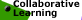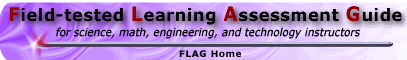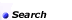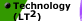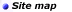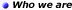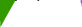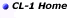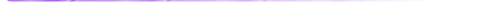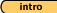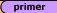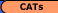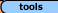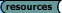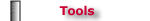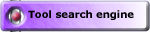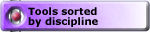## Tools - Math 'Plausible Estimation' Estimates for the USA Tasks, Set #4 (solutions)

Estimates for a Million, Set #1 (solutions)
Estimating for Amazing Facts: Set #2 (solutions) || Set #3 (solutions)
Estimates for the USA, Set #4 (solutions)

Malcolm Swan
Mathematics Education
University of Nottingham
Malcolm.Swan@nottingham.ac.uk

Jim Ridgway
School of Education
University of Durham
Jim.Ridgway@durham.ac.uk

 The aim of this assessment is to provide the opportunity for you to: develop a chain of reasoning that will enable you to make reasonable estimates of facts about the USA from population data communicate the assumptions upon which your estimate is based.

Try to estimate reasonable answers to each of the following questions.

Describe carefully at each stage any assumptions you make.

Show, step by step how you arrive at your estimate.

Each estimate should use the following fact:

The population of the USA is approximately 270 million.

(Note: Where possible the verification figures for these solutions have been quoted from Statistical Abstract of the US 1998, Hoover Business Press.)

1. Babies
 How many babies are born in the USA in each minute?Solution:

 Assumptions The population of the USA is approximately 270 million. Assume that the average life span is approximately 70 years. Assume the population is fairly stable. Assume that the distribution of the population is such that 1/70th of the population is 1 year old. Reasoning Then we may assume that 270/70 million babies are born each year.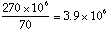babies per year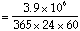babies per minute Answer: 7.4 babies per minute. (Verification: In 1996, 3,915,000 babies were born.)

1. Teachers
 How many K-8 Elementary School teachers are there in the USA?Solution:

 Assumptions The population of the USA is approximately 270 million. Approximately 1/8 of the population is in years K through 8. (If life span is approx 75 years and 9 of these are K-8, but fewer people are older) Assume an average of 25 children per class. Assume all teachers are working full-time. Reasoning Then there will beAnswer: 1.35 million Elementary teachers. (Verification: This estimate is a bit low. There are actually approximately 1.9 million.)

1. Newspapers
 How much is spent on newspapers in the USA each year?Solution:

 Assumptions The population of the USA is approximately 270 million. Assume that there are 3 people per household, on average. Assume that each household buys 1 paper per day. Assume that average cost of newspaper is \$0.60. Reasoning There are thus approximately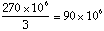Each year they will spend 90 x 106 x 365 x 0.60 = 20 x 109 dollars on newspaper. Answer: \$20 billion. (Verification: This estimate is a bit low. Actual figure is about 26 billion dollars.)

1. Cars
 How many cars are bought each year in the USA?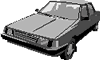Solution:

 Assumptions The population of the USA is approximately 270 million. Assume one car is owned per household. Assume that there are 3 people per household. Assume that life expectancy of a car is about 10 years. Assume that 1/10 of cars are replaced each year by new ones. Reasoning Then there are approximatelycars on the road in the US. If we divide this figure by 10, we can see that approximately 9 million cars are bought each year. Answer: 9 million. (Verification: In 1997, the actual figure was approximately 8 million.)

1. Dentists
 How many dentists are there in the USA?Solution:

 Assumptions The population of the USA is approximately 270 million. Each member of population sees dentist on average twice per year. Each consultation lasts about 20 minutes. Dentist sees patients for about 6 hours per day. Dentist works for 45 weeks per year. Reasoning Then, dentist can have 45 x 5 x 6 x 3 = 4,050 consultations per year. Number of consultations required = 540 million 540 million ÷ 4,050 = 130 thousand Answer: About 130 thousand dentists are required. (Verification: There are 160 thousand active dentists, or one dentist for every 1,600 of the population.)

Estimates for a Million, Set #1 (solutions)
Estimating for Amazing Facts: Set #2 (solutions) || Set #3 (solutions)
Estimates for the USA, Set #4 (solutions)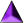Introduction || Assessment Primer || Matching Goals to CATs || CATs || Tools || Resources

Search || Who We Are || Site Map || Meet the CL-1 Team || WebMaster || Copyright || Download
College Level One (CL-1) Home || Collaborative Learning || FLAG || Learning Through Technology || NISE